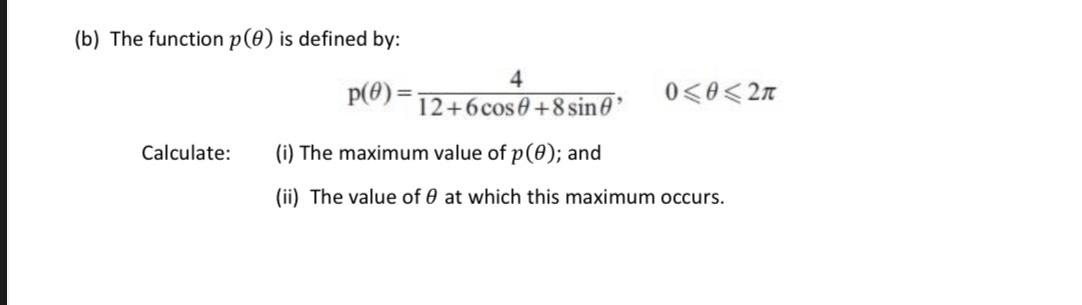### Still have math questions?

Algebra
Question(b) The function $$p ( \theta )$$ is defined by:

$$p ( \theta ) = \frac { 4 } { 12 + 6 \cos \theta + 8 \sin \theta } , 0 \leq \theta \leq 2 \pi$$

Calculate: (i) The maximum value of $$p ( \theta ) ;$$

(ii) The value of $$\theta$$ at which this maximum occurs.

$$\theta= \pi- arctan\frac{3}{4}= 143\degree\\p= \frac{1}{3}$$